# Problem

https://leetcode.com/problems/largest-sum-of-averages/description/

We partition a row of numbers A into at most K adjacent (non-empty) groups, then our score is the sum of the average of each group. What is the largest score we can achieve?

Note that our partition must use every number in A, and that scores are not necessarily integers.

Example:
Input:
A = [9,1,2,3,9]
K = 3
Output: 20
Explanation:
The best choice is to partition A into , [1, 2, 3], . The answer is 9 + (1 + 2 + 3) / 3 + 9 = 20.
We could have also partitioned A into [9, 1], , [3, 9], for example.
That partition would lead to a score of 5 + 2 + 6 = 13, which is worse.


Note:

• 1 <= A.length <= 100.
• 1 <= A[i] <= 10000.
• 1 <= K <= A.length.
• Answers within 10^-6 of the correct answer will be accepted as correct.

# Idea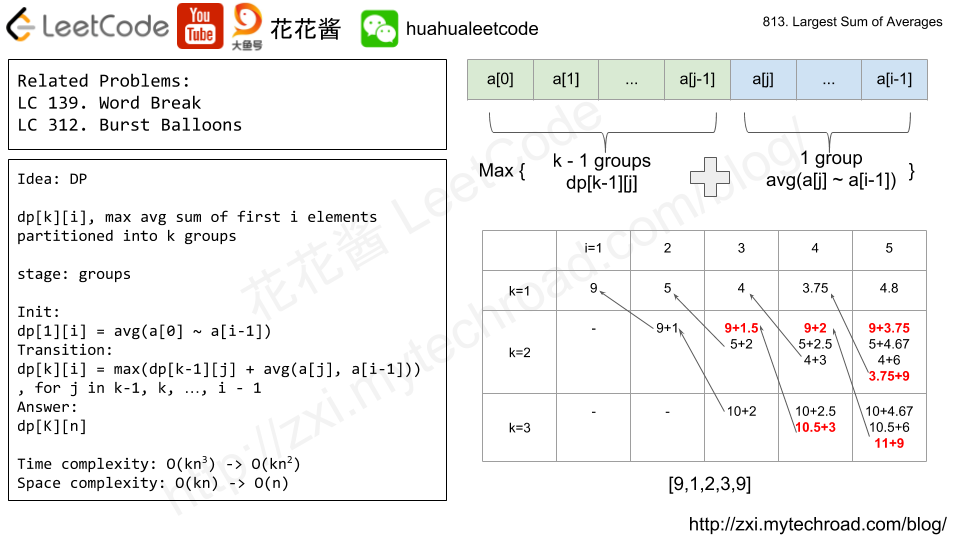DP, use dp[k][i] to denote the largest average sum of partitioning first i elements into k groups.

### Init

dp[i] = sum(a ~ a[i – 1]) / i, for i in 1, 2, … , n.

### Transition

dp[k][i] = max(dp[k – 1][j] + sum(a[j] ~ a[i – 1]) / (i – j)) for j in k – 1,…,i-1.

that is find the best j such that maximize dp[k][i]

largest sum of partitioning first j elements (a ~ a[j – 1]) into k – 1 groups (already computed)

+ average of a[j] ~ a[i – 1] (partition a[j] ~ a[i – 1] into 1 group).

dp[K][n]

# Solution 1: DP

Time complexity: O(kn^2)

Space complexity: O(kn)

# Problem:

https://leetcode.com/problems/binary-tree-pruning/description/

We are given the head node root of a binary tree, where additionally every node’s value is either a 0 or a 1.

Return the same tree where every subtree (of the given tree) not containing a 1 has been removed.

(Recall that the subtree of a node X is X, plus every node that is a descendant of X.)

Example 1:
Input: [1,null,0,0,1]
Output: [1,null,0,null,1]

Explanation:
Only the red nodes satisfy the property "every subtree not containing a 1".
The diagram on the right represents the answer.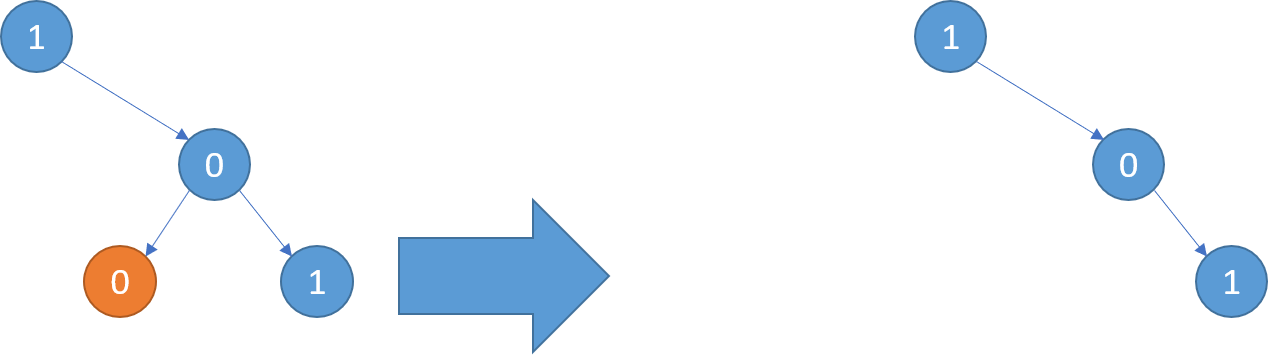Example 2:
Input: [1,0,1,0,0,0,1]
Output: [1,null,1,null,1]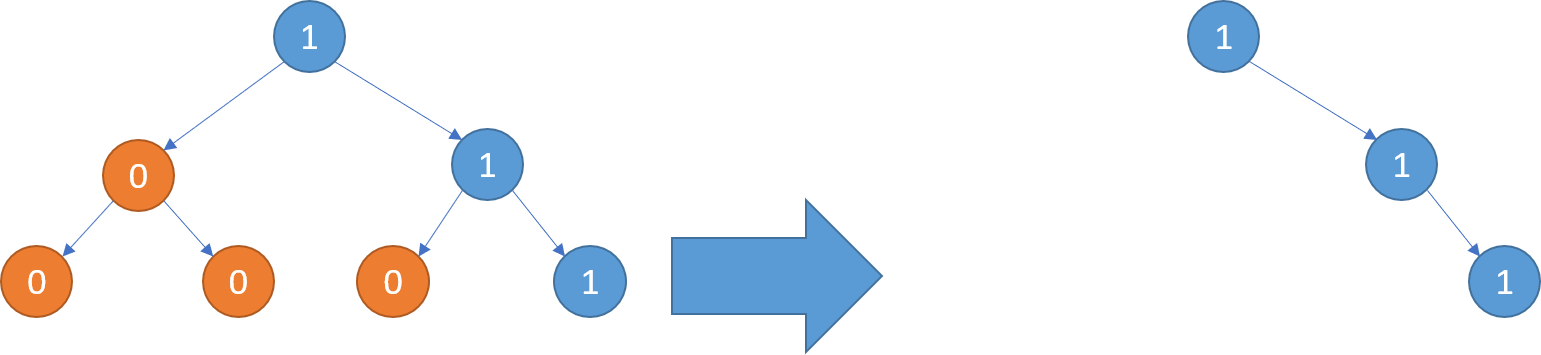Example 3:
Input: [1,1,0,1,1,0,1,0]
Output: [1,1,0,1,1,null,1]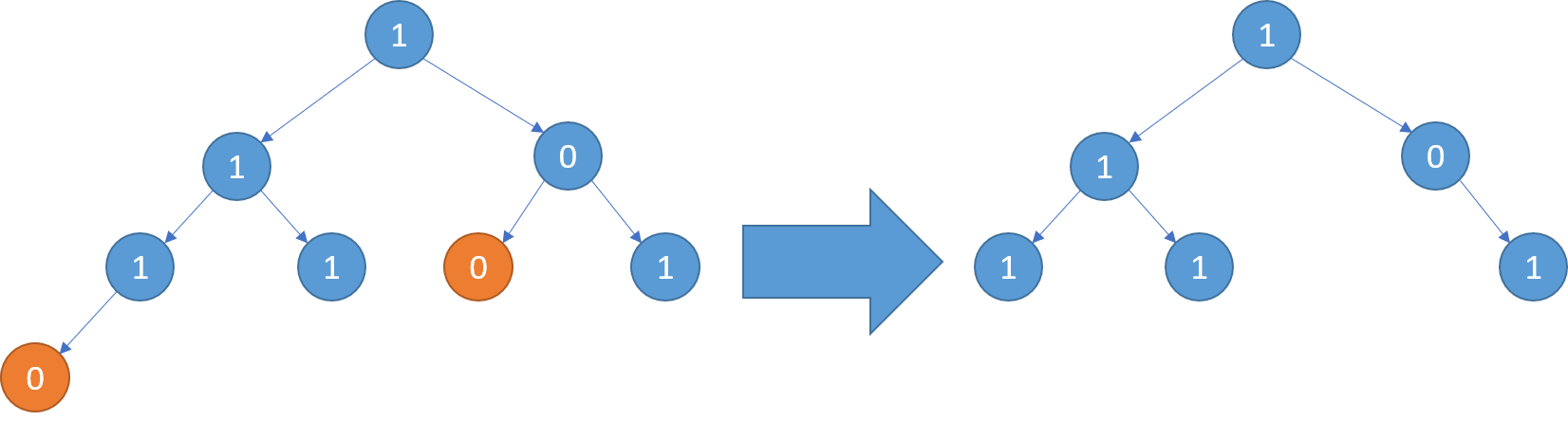Note:

• The binary tree will have at most 100 nodes.
• The value of each node will only be 0 or 1.

# Solution: Recursion

Time complexity: O(n)

Space complexity: O(h)

C++

Java

Python3

# Problem

In a 2 dimensional array grid, each value grid[i][j] represents the height of a building located there. We are allowed to increase the height of any number of buildings, by any amount (the amounts can be different for different buildings). Height 0 is considered to be a building as well.

At the end, the “skyline” when viewed from all four directions of the grid, i.e. top, bottom, left, and right, must be the same as the skyline of the original grid. A city’s skyline is the outer contour of the rectangles formed by all the buildings when viewed from a distance. See the following example.

What is the maximum total sum that the height of the buildings can be increased?

Example:
Input: grid = [[3,0,8,4],[2,4,5,7],[9,2,6,3],[0,3,1,0]]
Output: 35
Explanation:
The grid is:
[ [3, 0, 8, 4],
[2, 4, 5, 7],
[9, 2, 6, 3],
[0, 3, 1, 0] ]

The skyline viewed from top or bottom is: [9, 4, 8, 7]
The skyline viewed from left or right is: [8, 7, 9, 3]

The grid after increasing the height of buildings without affecting skylines is:

gridNew = [ [8, 4, 8, 7],
[7, 4, 7, 7],
[9, 4, 8, 7],
[3, 3, 3, 3] ]



Notes:

• 1 < grid.length = grid.length <= 50.
• All heights grid[i][j] are in the range [0, 100].
• All buildings in grid[i][j] occupy the entire grid cell: that is, they are a 1 x 1 x grid[i][j] rectangular prism.

# Solution: Greedy

Find the max of each row and column, increase the height of building at i,j to min(max_row[i], max_col[j]).

Time Complexity: O(m*n)

Space complexity: O(m+n)

C++

# Problem

https://leetcode.com/problems/subarray-sum-equals-k/description/

Given an array of integers and an integer k, you need to find the total number of continuous subarrays whose sum equals to k.

Example 1:

Input:nums = [1,1,1], k = 2
Output: 2


Note:

1. The length of the array is in range [1, 20,000].
2. The range of numbers in the array is [-1000, 1000] and the range of the integer k is [-1e7, 1e7].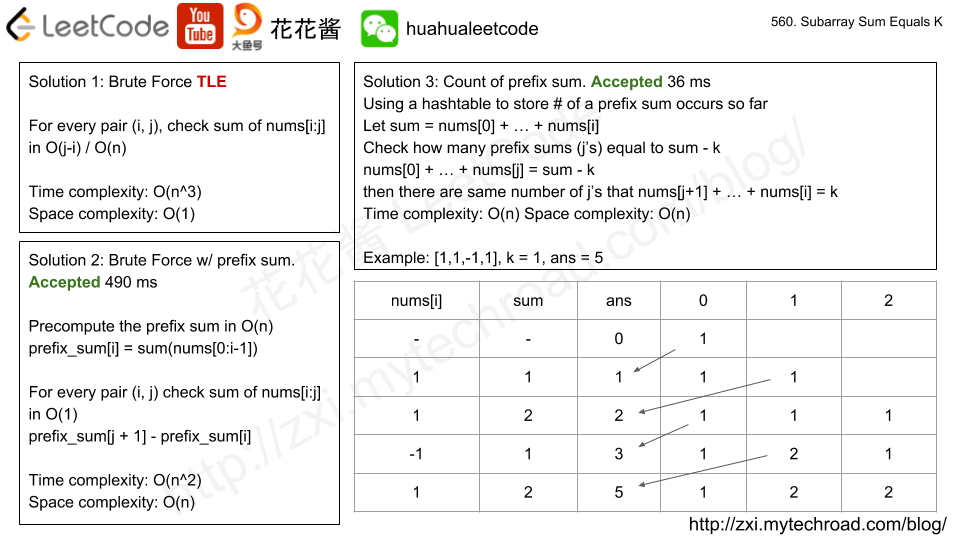# Solution -1: Brute Force

For every pair of i,j, check sum(nums[i:j]) in O(j-i) = O(n)

Time complexity: O(n^3) TLE

Space complexity: O(1)

# Solution 0: Brute Force + Prefix sun

Precompute the prefix sum and check sum of nums[i:j] in O(1)

Time complexity: O(n^2)

Space complexity: O(n)

C++

# Solution 1: Running Prefix sum

Keep tracking the prefix sums and their counts.

s -> count: how many arrays nums[0:j] (j < i) that has sum of s

cur_sum = sum(nums[0:i])

check how many arrays nums[0:j] (j < i) that has sum (cur_sum – k)

then there are the same number of arrays nums[j+1: i] that have sum k.

Time complexity: O(n)

Space complexity: O(n)

C++

# Problem

https://leetcode.com/problems/brick-wall/description/

There is a brick wall in front of you. The wall is rectangular and has several rows of bricks. The bricks have the same height but different width. You want to draw a vertical line from the top to the bottom and cross the least bricks.

The brick wall is represented by a list of rows. Each row is a list of integers representing the width of each brick in this row from left to right.

If your line go through the edge of a brick, then the brick is not considered as crossed. You need to find out how to draw the line to cross the least bricks and return the number of crossed bricks.

You cannot draw a line just along one of the two vertical edges of the wall, in which case the line will obviously cross no bricks.

Example:

Input:
[[1,2,2,1],
[3,1,2],
[1,3,2],
[2,4],
[3,1,2],
[1,3,1,1]]
Output: 2
Explanation:Note:

1. The width sum of bricks in different rows are the same and won’t exceed INT_MAX.
2. The number of bricks in each row is in range [1,10,000]. The height of wall is in range [1,10,000]. Total number of bricks of the wall won’t exceed 20,000.

# Solution: HashTable

Count boundaries, cut at the location with most common boundaries.

Time complexity: O(|bricks|)

Space complexity: O(|bricks|)

C++

Mission News Theme by Compete Themes.